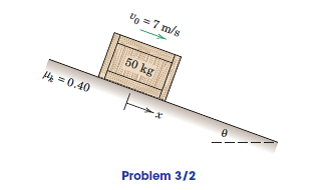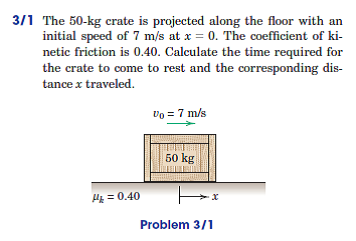Problem

# The 50-kg crate of Prob. 3/1 is now projected down an incline as shown with an initial s...

The 50-kg crate of Prob. 3/1 is now projected down an incline as shown with an initial speed of 7 m/s. Investigate the time t required for the crate to come to rest and the corresponding distance x traveled if (a) θ = 150 and (b) θ = 300.#### Step-by-Step Solution

Solution 1

Balancing the forces in the perpendicular direction of motion (y-Coordinate direction), it is obtained:

$$N=m g \cos \theta$$

Again balancing the forces in the direction of motion (x-Coordinate direction), it is obtained:

\begin{aligned} &m a=\mu_{k} N-m g \sin \theta \\ &m a=\mu_{k} m g \cos \theta-m g \sin \theta \\ &a=\mu_{k} g \cos \theta-g \sin \theta \end{aligned}

Here $$a$$ is the acceleration, $$g$$ is acceleration due to gravity, $$\mu_{k}$$ is the coefficient of kinetic friction and $$\theta$$ is inclination angle.

Substitute $$9.81 \mathrm{~m} / \mathrm{s}^{2}$$ for $$g, 0.4$$ for $$\mu_{k}$$ and $$15^{\circ}$$ for $$\theta=15^{\circ}$$ in above relation.

\begin{aligned} a &=9.81 \times\left(0.4 \times \cos 15^{\circ}-\sin 15^{\circ}\right) \\ &=1.2513 \mathrm{~m} / \mathrm{s}^{2} \end{aligned}

Calculate the distance travelled by using kinematic equation.

\begin{aligned} &v_{0}^{2}=2 a x \\ &x=\frac{v_{0}^{2}}{2 a} \end{aligned}

Here $$v_{0}$$ is the initial speed and $$a$$ is the acceleration.

Substitute $$7 \mathrm{~m} / \mathrm{s}$$ for $$v_{0}$$ and $$1.2513 \mathrm{~m} / \mathrm{s}^{2}$$ for $$a$$ in above equation.

\begin{aligned} x &=\frac{7^{2}}{2 \times 1.2513} \\ &=19.5799 \mathrm{~m} \end{aligned}

Therefore, distance travelled $$x$$ is $$19.6 \mathrm{~m}$$.

Calculate time required for the crate to come to rest.

\begin{aligned} &v_{0}=a t \\ &t=\frac{v_{0}}{a} \end{aligned}

Substitute $$7 \mathrm{~m} / \mathrm{s}$$ for $$v_{0}$$ and $$1.2513 \mathrm{~m} / \mathrm{s}^{2}$$ for $$a$$ in above equation.

\begin{aligned} t &=\frac{7}{1.2513} \\ &=5.594 \mathrm{~s} \end{aligned}

Therefore, time required for the crate to come to rest is $$5.59 \mathrm{~s}$$.

(b) Again, for $$\theta=30^{\circ}$$, it is obtained that:

\begin{aligned} a &=\mu_{k} g \cos \theta-g \sin \theta \\ &=9.81 \times\left(0.4 \times \cos 30^{\circ}-\sin 30^{\circ}\right) \\ &=-1.51 \frac{\mathrm{m}}{\mathrm{s}^{2}} \end{aligned}

As this acceleration is negative, the crate will move downwards and will not eventually come to rest.